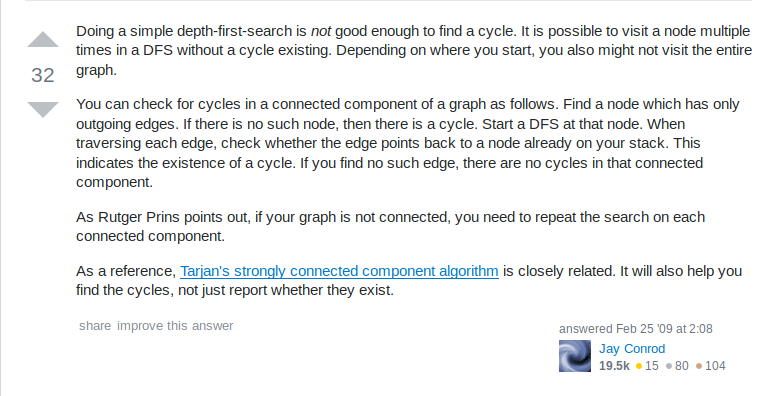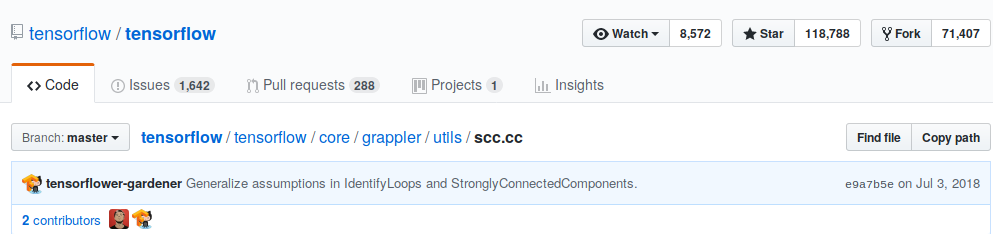@vbhvsgr

# All Git commit graphs are DAGs

## Here’s a DAG## Here’s a not-DAG# How???

## Topological sortSource: https://stackoverflow.com/questions/583876/how-do-i-check-if-a-directed-graph-is-acyclic

## Topological sort## SCCsSource: https://stackoverflow.com/questions/583876/how-do-i-check-if-a-directed-graph-is-acyclic

## SCCs## Tarjan’s Algorithm

`````` algorithm tarjan is
input: graph G = (V, E)
output: set of strongly connected components (sets of vertices)

index := 0
S := empty array
for each v in V do
if (v.index is undefined) then
strongconnect(v)
end if
end for

function strongconnect(v)
// Set the depth index for v to the smallest unused index
v.index := index
index := index + 1
S.push(v)
v.onStack := true

// Consider successors of v
for each (v, w) in E do
if (w.index is undefined) then
// Successor w has not yet been visited; recurse on it
strongconnect(w)
else if (w.onStack) then
// Successor w is in stack S and hence in the current SCC
// If w is not on stack, then (v, w) is a cross-edge in the DFS tree and must be ignored
// Note: The next line may look odd - but is correct.
// It says w.index not w.lowlink; that is deliberate and from the original paper
end if
end for

// If v is a root node, pop the stack and generate an SCC
if (v.lowlink = v.index) then
start a new strongly connected component
repeat
w := S.pop()
w.onStack := false
add w to current strongly connected component
while (w != v)
output the current strongly connected component
end if
end function``````

# Demonstration• stack: []
• output: []• stack: [ 1 ]
• output: []• stack: [ 2, 1 ]
• output: []• stack: [ 3, 2, 1 ]
• output: []• stack: [ 3, 2, 1 ]
• output: []• stack: [ 3, 2, 1 ]
• output: []• stack: [ 3, 2, 1 ]
• output: []• stack: []
• output: [ [3, 2, 1] ]• stack: [ 4 ]
• output: [ [3, 2, 1] ]• stack: [ 4 ]
• output: [ [3, 2, 1] ]• stack: [ 4 ]
• output: [ [3, 2, 1] ]• stack: [ 5, 4 ]
• output: [ [3, 2, 1] ]• stack: [ 5, 4 ]
• output: [ [3, 2, 1] ]• stack: [ 6, 5, 4 ]
• output: [ [3, 2, 1] ]• stack: [ 6, 5, 4 ]
• output: [ [3, 2, 1] ]• stack: [ 7, 6, 5, 4 ]
• output: [ [3, 2, 1] ]• stack: [ 7, 6, 5, 4 ]
• output: [ [3, 2, 1] ]• stack: [ 7, 6, 5, 4 ]
• output: [ [3, 2, 1] ]• stack: [ 7, 6, 5, 4 ]
• output: [ [3, 2, 1] ]• stack: [ 5, 4 ]
• output: [ [3, 2, 1], [ 7, 6 ] ]• stack: [ 5, 4 ]
• output: [ [3, 2, 1], [ 7, 6 ] ]• stack: [ 5, 4 ]
• output: [ [3, 2, 1], [ 7, 6 ] ]• stack: []
• output: [ [3, 2, 1], [ 7, 6 ], [ 5, 4 ] ]• stack: [ 8 ]
• output: [ [3, 2, 1], [ 7, 6 ], [ 5, 4 ] ]• stack: [ 8 ]
• output: [ [3, 2, 1], [ 7, 6 ], [ 5, 4 ] ]• stack: []
• output: [ [3, 2, 1], [ 7, 6 ], [ 5, 4 ], [ 8 ] ]

# Applications

## Dataflow processing